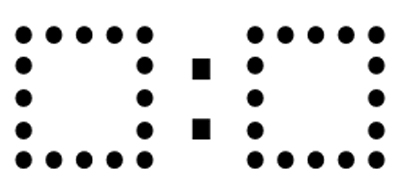# Equivalent Ratios

Directions: Using the digits 1 to 9, fill in the boxes to make as many ratios as you can that are equivalent to 2:3.### Hint

What makes a ratio equivalent?

There are 3 ratios that are equivalent to 2:3 including: 2:3, 4:6, and 6:9.

Source: Robert Kaplinsky

## Two Step Inequality with Fractional Coeffcient

Directions: Using the digits 1 to 7 at most one time each, place a digit …

1.It interesting, because I see this challenge as less difficult than the one you have for grade 6 involving creating three equivalent ratios for the numbers 1-9. As long as students know the scale factors, they could fairly easily solve this one, I think. Any experiences in the field on this one?

•2.